﻿ 基于Prophet-XGBoost模型的GNSS高程时间序列预测文章快速检索 高级检索
 大地测量与地球动力学2022, Vol. 42Issue (9): 898-903  DOI: 10.14075/j.jgg.2022.09.004### 引用本文LU Tieding, LI Zhen. Prediction of GNSS Vertical Coordinate Time Series Based on Prophet-XGBoost Model[J]. Journal of Geodesy and Geodynamics, 2022, 42(9): 898-903.### Foundation support

National Natural Science Foundation of China, No. 42061077, 42064001, 42104023;Natural Science Foundation of Jiangxi Province, No. 20202BAB214029.

### Corresponding author

LI Zhen, postgraduate, majors in GNSS data processing, E-mail: lizhenhd@163.com.

### About the first author

LU Tieding, professor, majors in surveying and mapping data processing, E-mail: tdlu@whu.edu.cn.

### 文章历史

1. 东华理工大学测绘工程学院，南昌市广兰大道418号，330013

1 模型原理与评价指标 1.1 XGBoost模型

XGBoost作为一种集成学习算法，可通过构建多个CART树对数据集进行预测，然后集成多个树模型的预测结果，从而输出最终预测数据。与同属于树模型算法的GBDT算法相比，XGBoost算法更为高效，并且在算法方面也进行过改进。XGBoost使用二阶泰勒展开式逼近目标函数的泛化误差部分，可有效简化目标函数的计算；XGBoost还可通过在目标函数中加入正则项来降低模型预测的波动性以及改善模型过拟合现象

 $\hat{y}_i=\varphi\left(x_i\right)=\sum\limits_{k=1}^K f_k\left(x_i\right)$ (1)

 $L(\varphi)=\sum\limits_i l\left(y_i, \hat{y}_i\right)+\sum\limits_k \varOmega\left(f_k\right)$ (2)

 $\varOmega\left(f_k\right)=\gamma T+\frac{1}{2} \lambda\|w\|^2$ (3)

XGBoost算法采用迭代法最小化目标函数。模型通过增加fj项在第j次迭代后得到减小的目标函数：

 $L^j=\sum\limits_{i=1}^n l\left(y_i, \hat{y}_i^{(j-1)}+f_j\left(x_i\right)\right)+\varOmega\left(f_j\right)$ (4)

 $\begin{gathered} L_{\text {split }}= \\ \frac{1}{2}\left[\frac{\left(\sum\limits_{i \in I_L} g_i\right)^2}{\sum\limits_{i \in I_L} h_i+\lambda}+\frac{\left(\sum\limits_{i \in I_R} g_i\right)^2}{\sum\limits_{i \in I_R} h_i+\lambda}-\frac{\left(\sum\limits_{i \in I} g_i\right)^2}{\sum\limits_{i \in I} h_i+\lambda}\right]-\gamma \end{gathered}$ (5)

 $g_i=\partial_{\hat{y}_i^{(j-1)}} l\left(y_i, \hat{y}_i^{(j-1)}\right)$ (6)
 $h_i=\partial_{\hat{y}_i^{(j-1)}}^2 l\left(y_i, \hat{y}_i^{(j-1)}\right)$ (7)

1.2 Prophet模型

Prophet模型是一种基于加性模型的时间序列预测模型，由趋势项、周期项和假日项组成[14-15]，表达式为：

 $y(t)=g(t)+s(t)+h(t)+\varepsilon_t$ (8)

 $s(t)=\sum\limits_{n=1}^N\left(a_n \cos \left(\frac{2 \pi n t}{P}\right)+b_n \sin \left(\frac{2 \pi n t}{P}\right)\right)$ (9)

1.3 精度评价指标

 ${\mathrm{MAE}}=\frac{1}{n} \sum\limits_{i=1}^n\left|y_i-\hat{y}_i\right|$ (10)
 ${\mathrm{RMSE}}=\sqrt{\frac{1}{n} \sum\limits_{i=1}^n\left(y_i-\hat{y}_i\right)^2}$ (11)

 $\rho_{Y \hat{Y}}=\frac{E(Y \hat{Y})-E(Y) E(\hat{Y})}{\sigma_Y \sigma_{\hat{Y}}}$ (12)

2 数据与模型构建 2.1 实验数据表 1 数据基本情况 Tab. 1 Basic information of data
2.2 模型适用性检验表 2 不同模型精度对比 Tab. 2 Comparison of accuracy of different models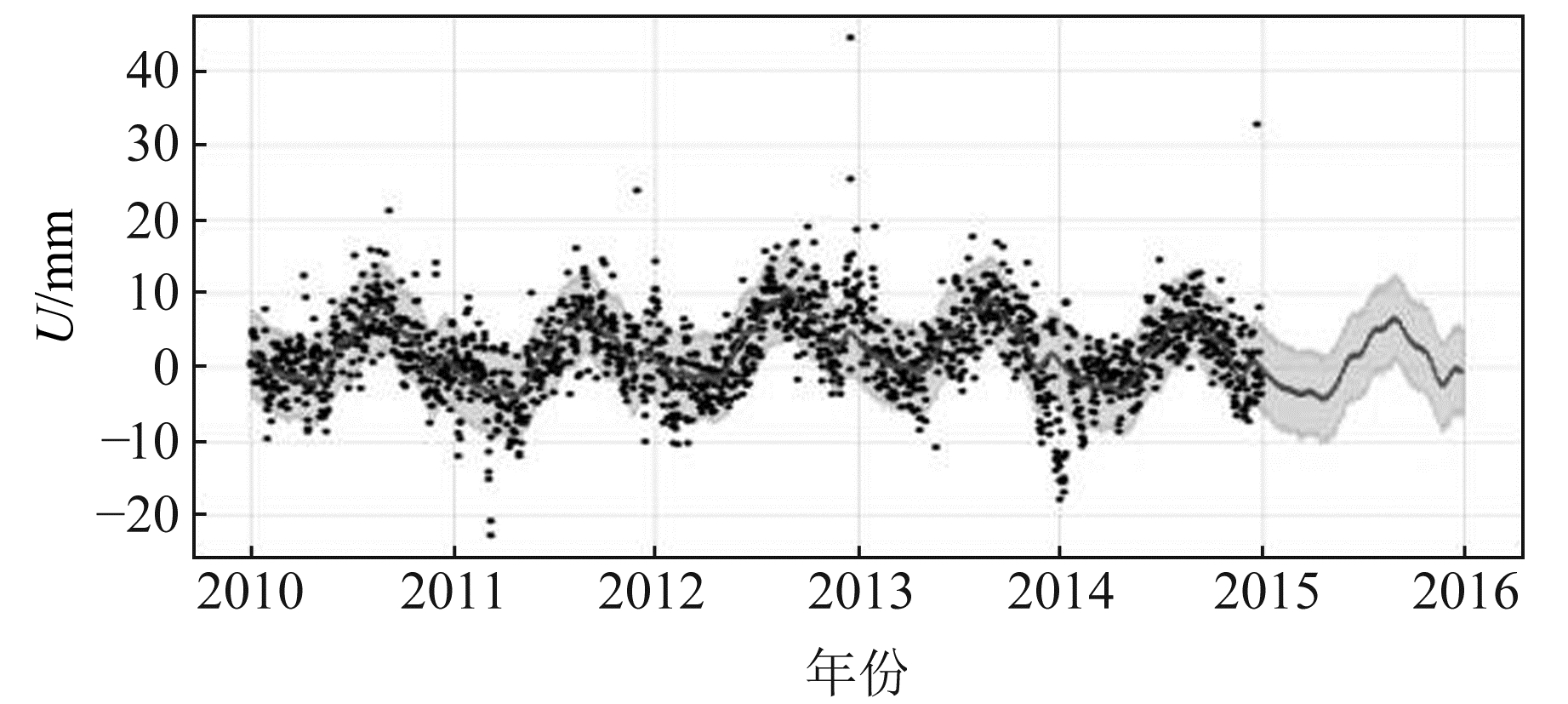图 1 ALGO站Prophet模型预测结果 Fig. 1 Prediction results of Prophet model of ALGO station
2.3 Prophet-XGBoost模型构建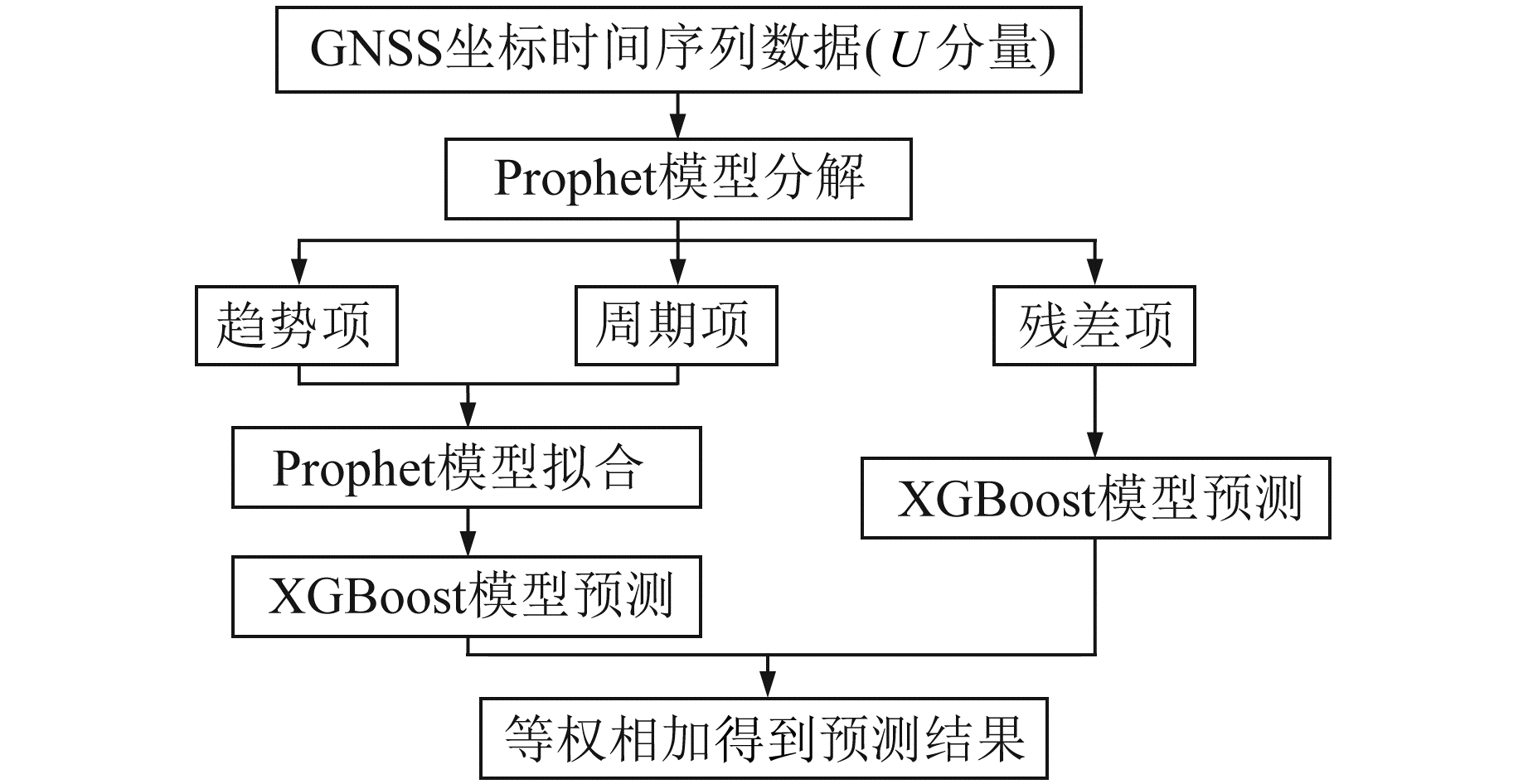图 2 Prophet-XGBoost模型构建流程 Fig. 2 Construction process of Prophet-XGBoost model

Prophet-XGBoost模型实现流程如下：

1) 通过Prophet模型对GNSS坐标时间序列U方向数据进行分解，将时间序列分解为趋势项、周期项和残差项，其中周期项由年周期项和周周期项等权相加构成。图 3为ALGO站Prophet模型分解结果。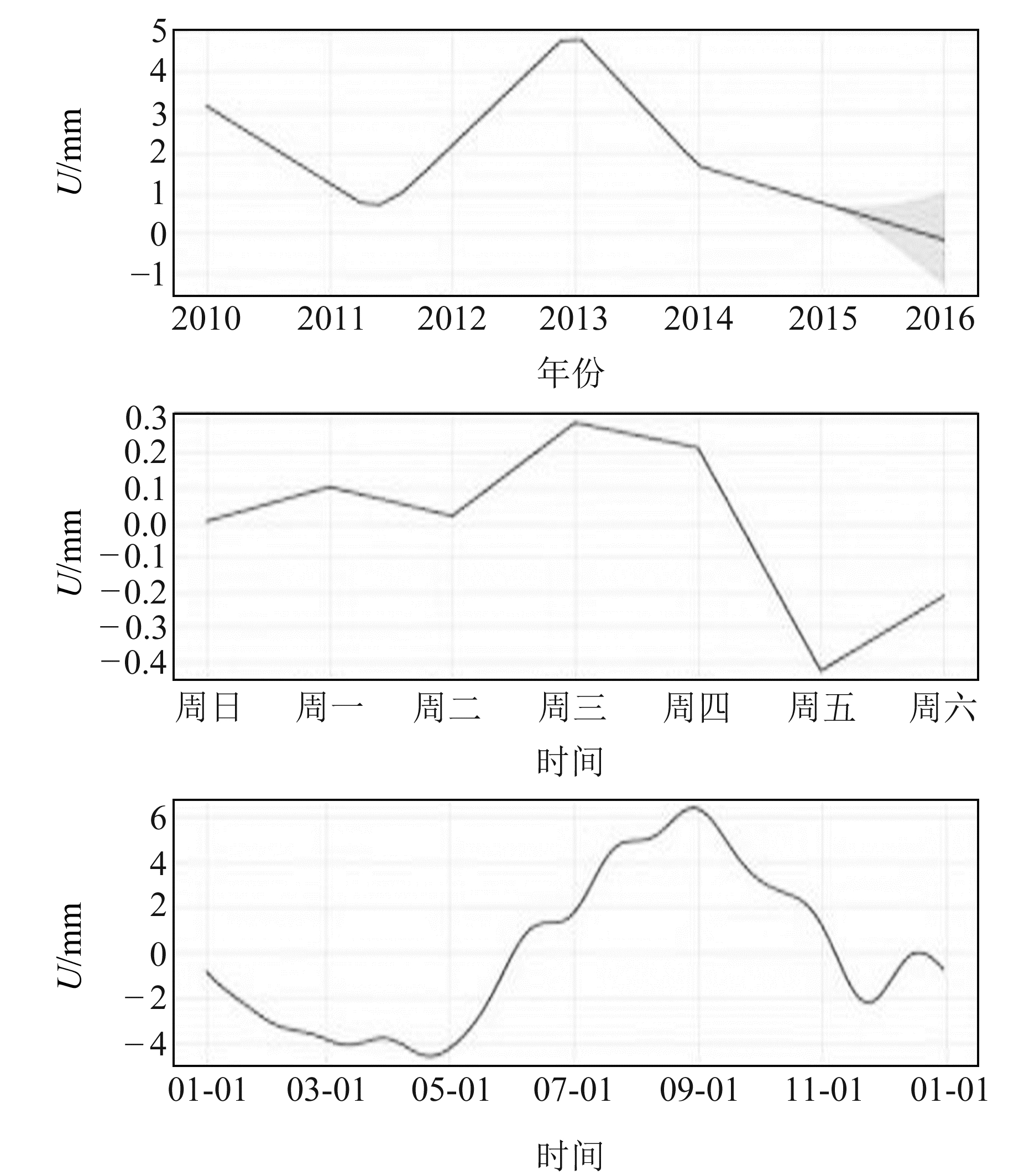图 3 ALGO站Prophet模型分解结果 Fig. 3 Decomposition results of Prophet model of ALGO station

2) 通过Prophet模型对分解出的趋势项和周期项进行拟合，然后使用XGBoost模型对拟合时间序列进行预测，得到预测值yfit图 4为ALGO站XGBoost模型拟合时间序列预测结果。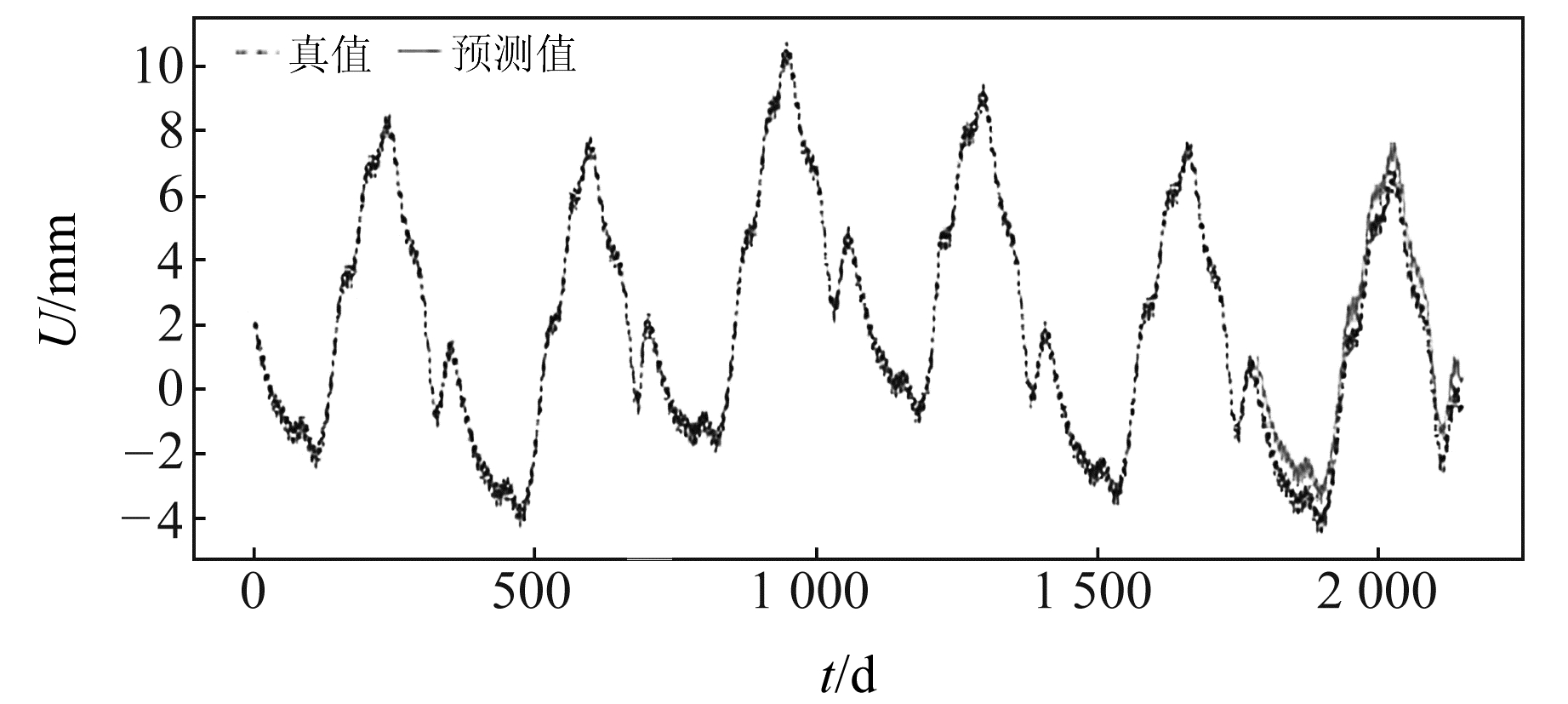图 4 ALGO站XGBoost模型拟合时间序列预测结果 Fig. 4 Prediction results of fitting time series of XGBoost model of ALGO station

3) 使用XGBoost模型对Prophet模型分解时间序列过程中遗留的残差项进行预测，得到预测值yresidual

4) 将两个预测结果等权相加，得到预测值ypred

 $y_{\text {pred }}=y_{\text {fit }}+y_{\text {residual }}$ (13)
2.4 实验结果分析表 3 预测精度对比 Tab. 3 Comparison of prediction accuracy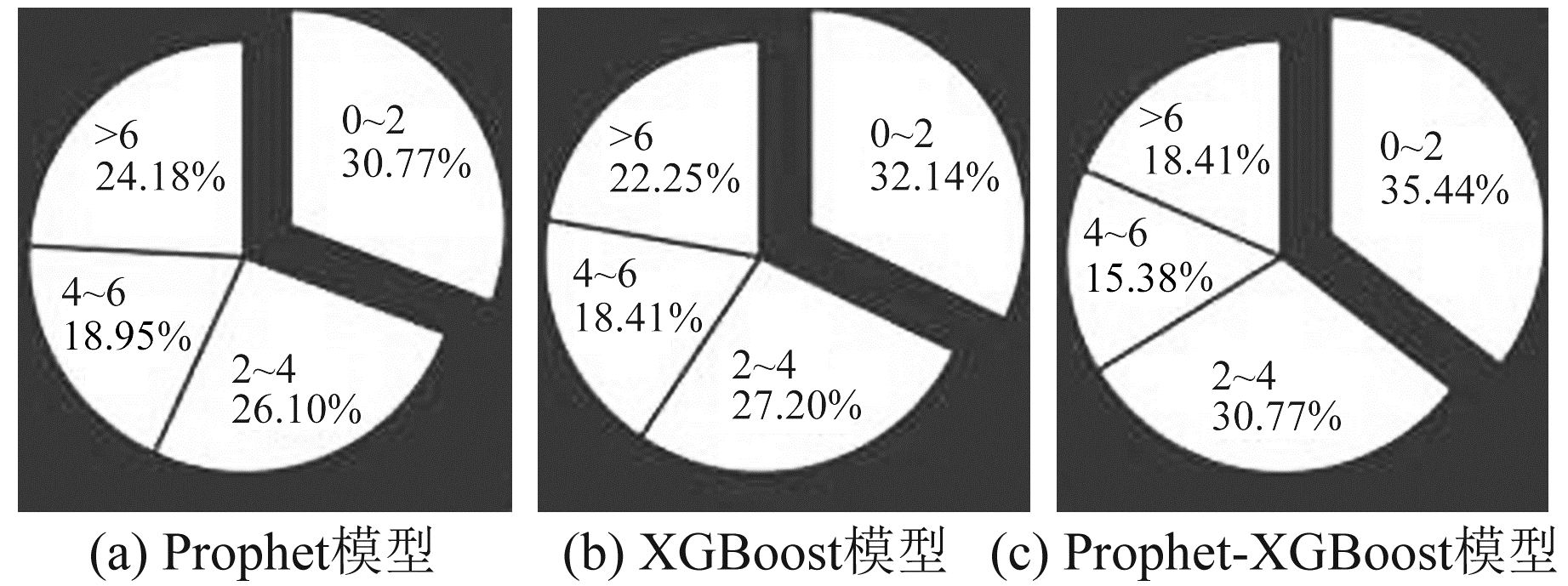图 5 各模型预测结果绝对误差分布 Fig. 5 Distribution of absolute error of prediction results of each model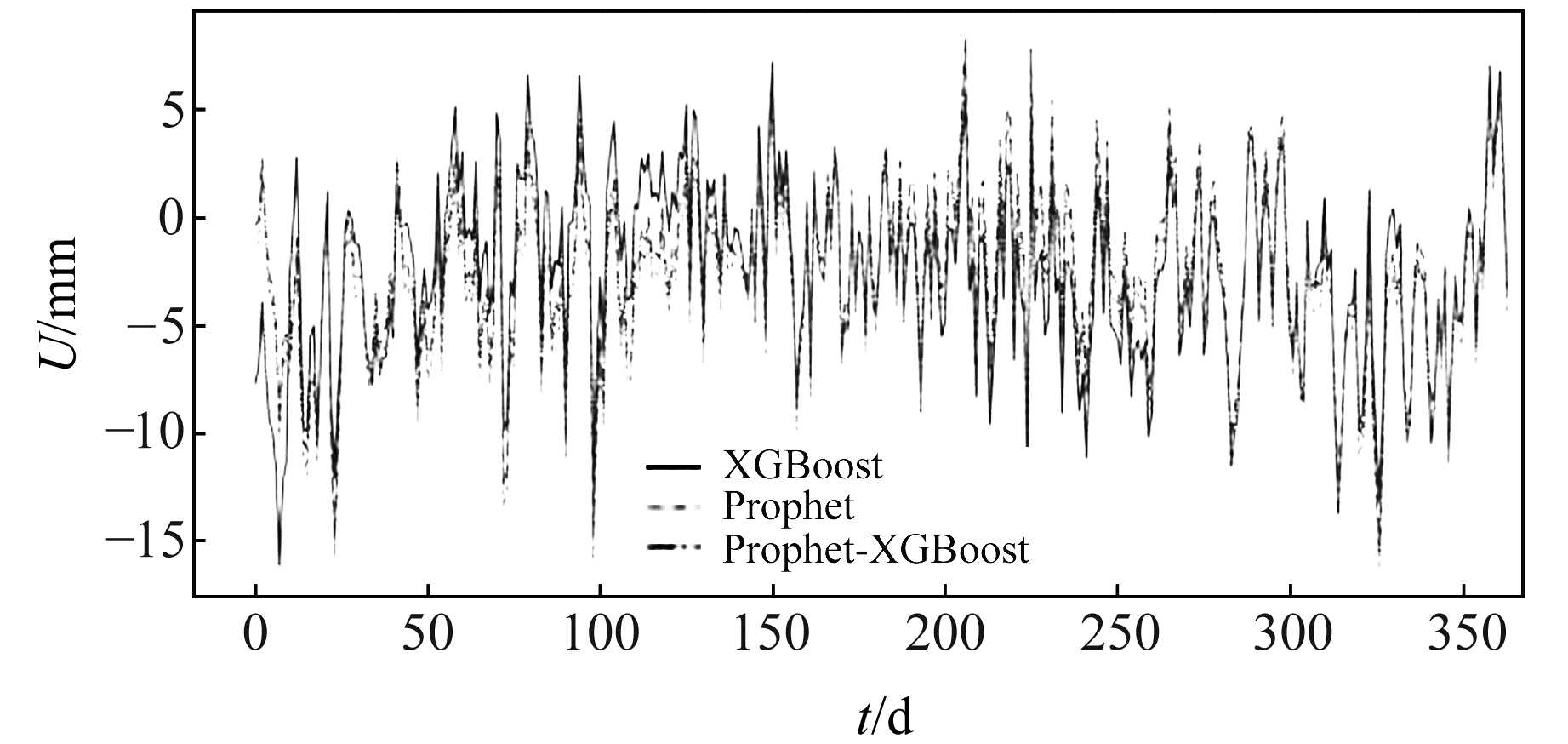图 6 模型预测结果误差对比 Fig. 6 Error comparison of prediction results of each model

3 结语

XGBoost模型和Prophet模型在实验过程中也存在需要改进之处：XGBoost模型对于GNSS坐标时间序列的趋势变化不够敏感以及Prophet模型对于GNSS坐标时间序列非线性部分预测能力较弱。同时考虑到XGBoost模型的特征项构建具有很大灵活性，研究人员可以使用非GNSS坐标时间序列数据辅助进行特征构造，如水文载荷对GNSS坐标时间序列U方向振幅具有较为显著的影响。因此，在以后研究中可以将水文载荷等因素纳入特征构造条件中，从而通过更多的特征子集进行模型构建和预测。基于该特性，Prophet-XGBoost模型可以作为通用预测模型，将其推广到其他研究领域，如海平面时间序列预测、天气预报和发病率预测，可极大增强该模型的适用性。

  姜卫平, 王锴华, 李昭, 等. GNSS坐标时间序列分析理论与方法及展望[J]. 武汉大学学报: 信息科学版, 2018, 43(12): 2 112-2 123 (Jiang Weiping, Wang Kaihua, Li Zhao, et al. Prospect and Theory of GNSS Coordinate Time Series Analysis[J]. Geomatics and Information Science of Wuhan University, 2018, 43(12): 2 112-2 123) (0)  Blewitt G, Lavallée D. Effect of Annual Signals on Geodetic Velocity[J]. Journal of Geophysical Research: Solid Earth, 2002, 107(B7) (0)  Deng L S, Jiang W P, Li Z, et al. Assessment of Second- and Third-Order Ionospheric Effects on Regional Networks: Case Study in China with Longer CMONOC GPS Coordinate Time Series[J]. Journal of Geodesy, 2017, 91(2): 207-227 DOI:10.1007/s00190-016-0957-y (0)  Wang J, Jiang W P, Li Z, et al. A New Multi-Scale Sliding Window LSTM Framework(MSSW-LSTM): A Case Study for GNSS Time-Series Prediction[J]. Remote Sensing, 2021, 13(16) (0)  Montillet J P, Williams S D P, Koulali A, et al. Estimation of Offsets in GPS Time-Series and Application to the Detection of Earthquake Deformation in the Far-Field[J]. Geophysical Journal International, 2015, 200(2): 1 207-1 221 DOI:10.1093/gji/ggu473 (0)  Xi R J, Jiang W P, Meng X L, et al. Rapid Initialization Method in Real-Time Deformation Monitoring of Bridges with Triple-Frequency BDS and GPS Measurements[J]. Advances in Space Research, 2018, 62(5): 976-989 DOI:10.1016/j.asr.2018.06.018 (0)  Chen Q S, Jiang W P, Meng X L, et al. Vertical Deformation Monitoring of the Suspension Bridge Tower Using GNSS: A Case Study of the Forth Road Bridge in the UK[J]. Remote Sensing, 2018, 10(3) (0)  Altamimi Z, Rebischung P, Métivier L, et al. ITRF2014: A New Release of the International Terrestrial Reference Frame Modeling Nonlinear Station Motions[J]. Journal of Geophysical Research: Solid Earth, 2016, 121(8): 6 109-6 131 DOI:10.1002/2016JB013098 (0)  Li Z, Chen W, Dam T, et al. Comparative Analysis of Different Atmospheric Surface Pressure Models and Their Impacts on Daily ITRF2014 GNSS Residual Time Series[J]. Journal of Geodesy, 2020, 94(4) (0)  贺小星, 花向红, 鲁铁定, 等. 时间跨度对GPS坐标序列噪声模型及速度估计影响分析[J]. 国防科技大学学报, 2017, 39(6): 12-18 (He Xiaoxing, Hua Xianghong, Lu Tieding, et al. Effect of Time Span on GPS Time Series Noise Model and Velocity Estimation[J]. Journal of National University of Defense Technology, 2017, 39(6): 12-18) (0)  李威, 鲁铁定, 贺小星, 等. 基于Prophet-RF模型的GNSS高程坐标时间序列预测分析[J]. 大地测量与地球动力学, 2021, 41(2): 116-121 (Li Wei, Lu Tieding, He Xiaoxing, et al. Prediction and Analysis of GNSS Vertical Coordinate Time Series Based on Prophet-RF Model[J]. Journal of Geodesy and Geodynamics, 2021, 41(2): 116-121) (0)  Tao R, Lu T D, Cheng Y M, et al. An Improved GNSS Vertical Time Series Prediction Model Using EWT[C]. China Satellite Navigation Conference, Nanchang, 2021 (0)  Pan B Y. Application of XGBoost Algorithm in Hourly PM2.5 Concentration Prediction[J]. IOP Conference Series: Earth and Environmental Science, 2018, 113 (0)  Taylor S J, Letham B. Forecasting at Scale[J]. The American Statistician, 2018, 72(1): 37-45 DOI:10.1080/00031305.2017.1380080 (0)  翟笃林, 张学民, 熊攀, 等. Prophet时序预测模型在电离层TEC异常探测中的应用[J]. 地震, 2019, 39(2): 46-62 (Zhai Dulin, Zhang Xuemin, Xiong Pan, et al. Detection of Ionospheric TEC Anomalies Based on Prophet Time-Series Forecasting Model[J]. Earthquake, 2019, 39(2): 46-62) (0)  陈志方. 中国货币政策的有效性评估——基于皮尔森相关系数的分析[J]. 中国商论, 2020(6): 48-49 (Chen Zhifang. The Effectiveness Evaluation of China's Monetary Policy-Analysis Based on Pearson Correlation Coefficient[J]. China Journal of Commerce, 2020(6): 48-49) (0)  He Y F, Nie G G, Wu S G, et al. Analysis and Discussion on the Optimal Noise Model of Global GNSS Long-Term Coordinate Series Considering Hydrological Loading[J]. Remote Sensing, 2021, 13(3) (0)
Prediction of GNSS Vertical Coordinate Time Series Based on Prophet-XGBoost Model
LU Tieding1LI Zhen11. Faculty of Geomatics, East China University of Technology, 418 Guanglan Road, Nanchang 330013, China
Abstract: According to the non-stationary and nonlinear characteristics of GNSS time series, we analyze the applicability and characteristics of XGBoost and Prophet models, and construct a Prophet-XGBoost prediction model. Firstly, using the Prophet model, we decompose the GNSS original time series, then carry out partial prediction by XGBoost model. We obtain prediction results by equal weight addition. We select the daily coordinate time series data of U component of ALGO, ALRT and BRST IGS stations in the experiment, and use MAE and RMSE as evaluation indexes. The experimental results show that compared with the single XGBoost model and Prophet model, the MAE and RMSE values of Prophet-XGBoost model are optimized to a certain extent. The effectiveness of this method is verified and can be used for GNSS time series prediction.
Key words: XGBoost model; Prophet model; time series; prediction